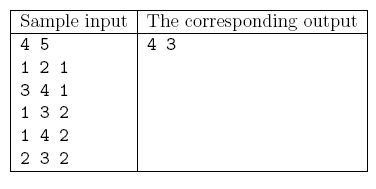Mathematical and Physical Journal
for High Schools
Issued by the MATFUND Foundation
 Already signed up? New to KöMaL?

#Problem S. 103. (December 2015)

S. 103. There are $\displaystyle N$ towns in a country. Your task is to design a road network such that one can travel between any two towns in exactly one way. The cost of building a particular road segment is directly proportional to its length, so we would like to minimize the total length of the network. However, there are also some hills in the country, making it impossible to build a road between any two arbitrary towns: it is only allowed to construct certain $\displaystyle M$ roads specified by the input data. Moreover, due to some technical restrictions, the construction company cannot build more than three roads of a given length, hence the network should be designed such that there are at most three roads of any given length. Your program should determine the number of ways the network with the minimum total length can be built.

Your program should read the values of $\displaystyle N$ and $\displaystyle M$ ($\displaystyle 1\le N \le 50\;000$, $\displaystyle 1\le M\le 200\;000$) from the first line of the standard input; then the $\displaystyle a_i$, $\displaystyle b_i$ and $\displaystyle c_i$ integers (separated by a space) from the following $\displaystyle M$ lines, representing the fact that a two-way road can be built from town $\displaystyle a_i$ to town $\displaystyle b_i$ with cost $\displaystyle c_i$ ($\displaystyle 1\le c_i\le 1\;000\;000$). The first and only line of the standard output should contain the minimum total length of a valid network, moreover, the remainder on division of the number of possible networks by $\displaystyle 1\;000\;000\;007$.Explanation: The minimum total length is achieved if we choose the edges of length 1 and one edge of length 2.

Scoring and bounds. You can get 1 point for a brief and proper documentation clearly describing your solution. Nine further points can be obtained provided that your program solves any valid input within 1 second of running time.

The source code of your program and a short documentation-also describing which developer environment to use for compiling the source-should be submitted in a compressed file s103.zip.

(10 pont)

Deadline expired on January 11, 2016.

### Statistics:

 14 students sent a solution. 10 points: Csenger Géza, Erdős Márton, Gáspár Attila, Noszály Áron. 5 points: 1 student. 3 points: 3 students. 2 points: 3 students. 0 point: 3 students.

Problems in Information Technology of KöMaL, December 2015